Expected value and moments (July 29, 2005). Category: Probability concepts

Someone asked me what a statistical moment is. That's a rather technical term and is not needed except in rather theoretical and mathematical discussions. But it is still worth defining. First, though, let me review what an expected value is:

Suppose we have a random variable X, which represents the number of girls in a family of three children. Without too much effort, you can compute the following probabilities.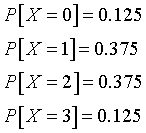The expected value of X, E[X], is computed asThis calculation can be easily generalized to more complicated situations. Suppose that a rich uncle plans to give you \$2,000 for each child in your family, with a bonus of \$500 for each girl. The formula for the bonus isWhat is your expected bonus?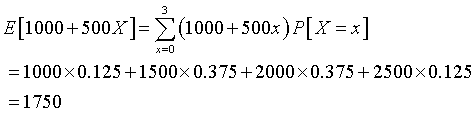Now, you could have calculated the same value by taking the expected number of children and plugging it into the equation.This is a rather simplistic example, but the concept of expected value does arise from time to time in real medical problems. For example, in a study of a novel test for bladder cancer,

six dogs were trained to discriminate between urine from patients with bladder cancer and urine from diseased and healthy controls and then evaluated in tests requiring the selection of one bladder cancer urine sample from six controls.

The success rate was quite good, 41%, especially when you compared it to

an expected value of 1 in 7 (14%)

Olfactory detection of human bladder cancer by dogs: proof of principle study. Willis CM, Church SM, Guest CM, Cook WA, McCarthy N, Bransbury AJ, Church MR, Church JC. Bmj 2004: 329(7468); 712. [Medline] [Abstract] [Full text] [PDF]

Quite often, a bit of intuition and common sense will sometimes help you calculate an expected value. Otherwise, you have to use a bit of mathematics. Most advanced applications of expected value require knowledge of infinite sums and/or integrals. If X is a continuous random variable with density function f(x), then the expected value of X isTo calculate such an expected value, you often have to recall your college Calculus formulas. Here are a few simple examples. An exponential distribution has a density functionwhere b is a scale parameter. You would compute the expected value asNotice that the lower limit of the integral is zero rather than -infinity because the density is defined only for values between 0 and infinity.

This integral is a bit tedious, but you can use integration by parts to compute it. The basic form for integration by parts isCareful selection of u and dv yields the following.When you evaluate this integral at the limits of zero and infinity, most of the terms become zero and you are left withNow that was a lot of work for a relatively simple expected value calculation. For most distributions, someone else has already done the Calculus for you and you can just consult the appropriate reference. One of my favorite books that has a lot of these expected value calculations for a wide range of statistical distributions is:

• Statistical Distributions Second Edition. Evans M, Hastings N, Peacock B (1993) New York: John Wiley & Sons. ISBN: 0471559512. [BookFinder4U link]

Moments

A moment is a special type of expected value. The formal definition of the nth moment isdepending on whether x is continuous or discrete. In simpler terms, a moment is the expect value of a power of x. A central moment is defined similarly, except you subtract the mean before raising to a power.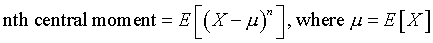Moments are used in the calculation of important statistics like the mean, variance, skewness, and kurtosis of a distribution. The mean is the first moment, the variance is the second central moment. The skewness and kurtosis are related to the third and fourth central moments..

The second moment of the exponential distribution, for example, isThis integral is again a bit tedious to calculate, because you have to use integration by parts twice. When you do this you getThe second central moment of the exponential distribution is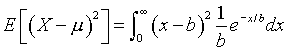Again, you can use Calculus, but there is a shortcut formula that provides an even simpler answer.Skewness is defined as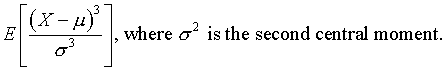Again, you need a bit of patience, but repeated integration by parts yieldsWhat does all this tell us from a practical perspective? First notice that the variance of the exponential distribution is equal to the square of the mean. This tells you that exponential distributions with large means also have large variances. The skewness is +2 which tells you that the distribution is not symmetric, but instead is positively skewed. This means that this distribution has a greater tendency to produce outliers on the high end rather than the low end of the distribution.This work is licensed under a Creative Commons Attribution 3.0 United States License. It was written by Steve Simon and was last modified on 04/01/2010.